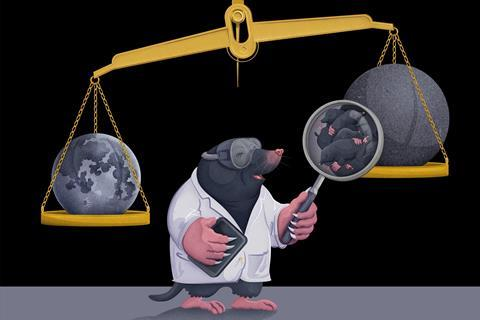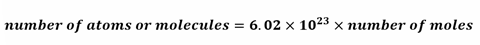Equip your students with the tools to dig themselves out of any difficulties with moles and Avogadro’s numberIn chemistry, a mole is a really big number. This number (6.02 x 1023) comes from the number of atoms in 12 g of carbon-12 (this is the carbon isotope with six protons and six neutrons).

So, we can say that one mole of protons has a mass of one gram, and one mole of neutrons has a mass of one gram, as protons and neutrons have similar masses.

This means that:

• One mole of 1 H atoms has a mass of one g.
• One mole of 19 F atoms has a mass of 19 g, and two moles have a mass of 38 g.
• One mole of NH3 molecules – which has a relative molecular mass (Mr) of 17 – has a mass of 17 g, and half a mole has a mass of 8.5 g.
• One mole of ibuprofen (C13 H18 O2) has a mass of 206 g, and 0.01 moles have a mass of 2.06 g (which is still way more than is in an ibuprofen tablet).

Moles allow us to compare the number of atoms or molecules in two or more different substances without writing out long numbers.

Infographic poster, fact sheet, worksheet and teacher notes. Display the poster in your classroom or on a projector. Alternatively, print it and use it as a handout.

Use the accompanying fact sheet and worksheet to get your students to apply their mathematical skills to three different types of problems involving moles.

## Calculating moles

The relationship between moles (mol), mass (g) and Mr (g mol-1) can be represented by this equation:### Did you know …?

The average furry European mole is approximately 100 g. So, a mole of furry European moles would have a mass of: 6.02 x 1022 kg. Similar to the mass of the Moon at 7.35 x 1022 kg. That is one huge ball of fur.

Remember that we said a mole is a really big number

We can use Avogadro’s constant to calculate the number of atoms or molecules from the number of moles or vice versa, using the following relationship:### Did you know…?

Amedeo Avogadro didn’t calculate the value of the mole, but he was the first to claim that different gases at the same volume and pressure would contain the same number of particles. Sadly, he died before anyone figured out the number that bears his name.

## More resources

• Use the ideas in these five steps to help learners master mole calculations.
• Quiz 16–18 learners on the mole, moles and mass and other chemistry methods with these quantitative chemistry questions.
• Practise more mathematical competencies with these Starter for 10 questions for learners transitioning to post-16 study.

• #### Introducing moles and Avogadro's number poster

Handout | PDF, Size 0.78 mb
• #### Introducing moles and Avogadro's number poster_white

Handout | PDF, Size 0.81 mb
• #### Moles calculations student sheet

Handout | PDF, Size 0.25 mb
• #### Moles calculations teacher notes

Handout | PDF, Size 0.23 mb
• #### Moles calculations student sheet

Editable handout | Word, Size 0.46 mb
• #### Moles calculations teacher notes

Editable handout | Word, Size 0.45 mb
• #### Introducing moles and Avogadro's number fact sheet

Editable handout | Word, Size 0.45 mb
• #### Introducing moles and Avogadro's number fact sheet

Handout | PDF, Size 0.17 mb